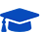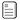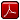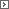Mathematics Adult Basic Education 2Course PrefacePrintable Version (pdf)Course Introduction

Core Standards of the Course

Standards for Mathematical Practice

MP.1
Make sense of problems and persevere in solving them.
Mathematically proficient students start by explaining to themselves the meaning of a problem and looking for entry points to its solution. They analyze givens, constraints, relationships, and goals. They make conjectures about the form and meaning of the solution and plan a solution pathway rather than simply jumping into a solution attempt. They consider analogous problems, and try special cases and simpler forms of the original problem in order to gain insight into its solution. They monitor and evaluate their progress and change course if necessary. Students might, depending on the context of the problem, transform algebraic expressions or change the viewing window on their graphing calculator to get the information they need. Mathematically proficient students can explain correspondences between equations, verbal descriptions, tables, and graphs or draw diagrams of important features and relationships, graph data, and search for regularity or trends. Less experienced students might rely on using concrete objects or pictures to help conceptualize and solve a problem. Mathematically proficient students check their answers to problems using a different method, and they continually ask themselves, "Does this make sense?" They can understand the approaches of others to solving complex problems and identify correspondences between different approaches.

MP.2
Reason abstractly and quantitatively.
Mathematically proficient students make sense of quantities and their relationships in problem situations. They bring two complementary abilities to bear on problems involving quantitative relationships: the ability to decontextualize-to abstract a given situation and represent it symbolically and manipulate the representing symbols as if they have a life of their own, without necessarily attending to their referents-and the ability to contextualize, to pause as needed during the manipulation process in order to probe into the referents for the symbols involved. Quantitative reasoning entails habits of creating a coherent representation of the problem at hand; considering the units involved; attending to the meaning of quantities, not just how to compute them; and knowing and flexibly using different properties of operations and objects.

MP.3
Construct viable arguments and critique the reasoning of others.
Mathematically proficient students understand and use stated assumptions, definitions, and previously established results in constructing arguments. They make conjectures and build a logical progression of statements to explore the truth of their conjectures. They are able to analyze situations by breaking them into cases, and can recognize and use counterexamples. They justify their conclusions, communicate them to others, and respond to the arguments of others. They reason inductively about data, making plausible arguments that take into account the context from which the data arose. Mathematically proficient students are also able to compare the effectiveness of two plausible arguments, distinguish correct logic or reasoning from that which is flawed, and-if there is a flaw in an argument-explain what it is. Less experienced students can construct arguments using concrete referents such as objects, drawings, diagrams, and actions. Such arguments can make sense and be correct, even though they are not generalized or made formal until later. Later, students learn determine domains to which an argument applies. Students at all levels can listen or read the arguments of others, decide whether they make sense, and ask useful questions to clarify or improve the arguments.

MP.4
Model with mathematics.
Mathematically proficient students can apply the mathematics they know to solve problems arising in everyday life, society, and the workplace. This might be as simple as writing an addition equation to describe a situation. A student might apply proportional reasoning to plan a school event or analyze a problem in the community. A student might use geometry to solve a design problem or use a function to describe how one quantity of interest depends on another. Mathematically proficient students who can apply what they know are comfortable making assumptions and approximations to simplify a complicated situation, realizing that these may need revision later. They are able to identify important quantities in a practical situation and map their relationships using such tools as diagrams, two-way tables, graphs, flowcharts and formulas. They can analyze those relationships mathematically to draw conclusions. They routinely interpret their mathematical results in the context of the situation and reflect on whether the results make sense, possibly improving the model if it has not served its purpose.

MP.5
Use appropriate tools strategically.
Mathematically proficient students consider the available tools when solving a mathematical problem. These tools might include pencil and paper, concrete models, a ruler, a protractor, a calculator, a spreadsheet, a computer algebra system, a statistical package, or dynamic geometry software. Proficient students are sufficiently familiar with tools appropriate for their course to make sound decisions about when each of these tools might be helpful, recognizing both the insight to be gained and their limitations. For example, mathematically proficient students analyze graphs of functions and solutions generated using a graphing calculator. They detect possible errors by strategically using estimation and other mathematical knowledge. When making mathematical models, they know that technology can enable them to visualize the results of varying assumptions, explore consequences, and compare predictions with data. Mathematically proficient students at various levels are able to identify relevant external mathematical resources, such as digital content located on a website, and use them to pose or solve problems. They are able to use technological tools to explore and deepen their understanding of concepts.

MP.6
Attend to precision.
Mathematically proficient students try to communicate precisely to others. They try to use clear definitions in discussion with others and in their own reasoning. They state the meaning of the symbols they choose, including using the equal sign consistently and appropriately. They are careful about specifying units of measure, and labeling axes to clarify the correspondence with quantities in a problem. They calculate accurately and efficiently, express numerical answers with a degree of precision appropriate for the problem context. Less experienced students give carefully formulated explanations to each other. By the time they reach high school they have learned to examine claims and make explicit use of definitions.

MP.7
Look for and make use of structure.
Mathematically proficient students look closely to discern a pattern or structure. Students, for example, might notice that three and seven more is the same amount as seven and three more, or they may sort a collection of shapes according to how many sides the shapes have. Later, students will see 7 � 8 equals the well-remembered 7 � 5 + 7 � 3, in preparation for learning about the distributive property. In the expression x2+ 9x + 14, students can see the 14 as 2 � 7 and the 9 as 2 + 7. They recognize the significance of an existing line in a geometric figure and can use the strategy of drawing an auxiliary line for solving problems. They also can step back for an overview and shift perspective. They can see complicated things, such as some algebraic expressions, as single objects or as being composed of several objects. For example, they can see 5 - 3(x - y)2 as 5 minus a positive number times a square and use that to realize that its value cannot be more than 5 for any real numbers x and y.

MP.8
Look for and express regularity in repeated reasoning.
Mathematically proficient students notice if calculations are repeated, and look both for general methods and for shortcuts. Early on, students might notice when dividing 25 by 11 that they are repeating the same calculations over and over again, and conclude they have a repeating decimal. By paying attention to the calculation of slope as they repeatedly check whether points are on the line through (1, 2) with slope 3, students might abstract the equation (y - 2)/(x - 1) = 3. Noticing the regularity in the way terms cancel when expanding (x-1)(x+1), (x-1)(x2+x+1), and (x-1)(x3 +x2+x+1) might lead them to the general formula for the sum of a geometric series. As they work to solve a problem, mathematically proficient students maintain oversight of the process, while attending to the details. They continually evaluate the reasonableness of their intermediate results.

Number and Operations: Base Ten

Understand place value.

2.NBT.1
Understand that the three digits of a three-digit number represent amounts of hundreds, tens, and ones; e.g., 706 equals 7 hundreds, 0 tens, and 6 ones. Understand the following as special cases:

1. 100 can be thought of as a bundle of ten tens - called a "hundred."
2. The numbers 100, 200, 300, 400, 500, 600, 700, 800, 900 refer to one, two, three, four, five, six, seven, eight, or nine hundreds (and 0 tens and 0 ones).

2.NBT.2
Count within 1000; skip-count by 5s, 10s, and 100s.

2.NBT.3
Read and write numbers to 1000 using base-ten numerals, number names, and expanded form.

2.NBT.4
Compare two three-digit numbers based on meanings of the hundreds, tens, and ones digits, using >, =, and < symbols to record the results of comparisons.

Use place value understanding and properties of operations to add and subtract.

2.NBT.6
Add up to four two-digit numbers using strategies based on place value and properties of operations.

2.NBT.7
Add and subtract within 1000, using concrete models or drawings and strategies based on place value, properties of operations, and/or the relationship between addition and subtraction; relate the strategy to a written method. Understand that in adding or subtracting three-digit numbers, one adds or subtracts hundreds and hundreds, tens and tens, ones and ones; and sometimes it is necessary to compose or decompose tens or hundreds.

2.NBT.8
Mentally add 10 or 100 to a given number 100-900, and mentally subtract 10 or 100 from a given number 100-900.

2.NBT.9
Explain why addition and subtraction strategies work, using place value and the properties of operations.

Use place value understanding and properties of operations to perform multi-digit arithmetic.13

3.NBT.1
Use place value understanding to round whole numbers to the nearest 10 or 100.

3.NBT.2
Fluently add and subtract within 1000 using strategies and algorithms based on place value, properties of operations, and/or the relationship between addition and subtraction.

3.NBT.3
Multiply one-digit whole numbers by multiples of 10 in the range 10-90 (e.g., 9 � 80, 5 � 60) using strategies based on place value and properties of operations.

Number and Operations: Fractions14

Develop understanding of fractions as numbers.

3.NF.1
Understand a fraction 1/b as the quantity formed by 1 part when a whole is partitioned into b equal parts; understand a fraction a/b as the quantity formed by a parts of size 1/b.

3.NF.2
Understand a fraction as a number on the number line; represent fractions on a number line diagram.

1. Represent a fraction 1/b on a number line diagram by defining the interval from 0 to 1 as the whole and partitioning it into b equal parts. Recognize that each part has size 1/b and that the endpoint of the part based at 0 locates the number 1/b on the number line. (3.NF.2a)
2. Represent a fraction a/b on a number line diagram by marking off a lengths 1/b from 0. Recognize that the resulting interval has size a/b and that its endpoint locates the number a/b on the number line. (3.NF.2b)

3.NF.3
Explain equivalence of fractions in special cases, and compare fractions by reasoning about their size.

1. Understand two fractions as equivalent (equal) if they are the same size, or the same point on a number line. (3.NF.3a)
2. Recognize and generate simple equivalent fractions, e.g., 1/2 = 2/4, 4/6 = 2/3. Explain why the fractions are equivalent, e.g., by using a visual fraction model. (3.NF.3b)
3. Express whole numbers as fractions, and recognize fractions that are equivalent to whole numbers. Examples: Express 3 in the form 3 = 3/1; recognize that 6/1 = 6; locate 4/4 and 1 at the same point of a number line diagram. (3.NF.3c)
4. Compare two fractions with the same numerator or the same denominator by reasoning about their size. Recognize that comparisons are valid only when the two fractions refer to the same whole. Record the results of comparisons with the symbols >, =, or <, and justify the conclusions, e.g., by using a visual fraction model. (3.NF.3d)

Operations and Algebraic Thinking

Represent and solve problems involving addition and subtraction.

2.OA.1
Use addition and subtraction within 100 to solve one- and two-step word problems involving situations of adding to, taking from, putting together, taking apart, and comparing, with unknowns in all positions, e.g., by using drawings and equations with a symbol for the unknown number to represent the problem.

2.OA.2
Fluently add and subtract within 20 using mental strategies. Know from memory all sums of two onedigit numbers.

Represent and solve problems involving multiplication and division.

3.OA.1
Interpret products of whole numbers, e.g., interpret 5 � 7 as the total number of objects in 5 groups of 7 objects each. For example, describe a context in which a total number of objects can be expressed as 5 � 7.

3.OA.2
Interpret whole-number quotients of whole numbers, e.g., interpret 56 � 8 as the number of objects in each share when 56 objects are partitioned equally into 8 shares, or as a number of shares when 56 objects are partitioned into equal shares of 8 objects each. For example, describe a context in which a number of shares or a number of groups can be expressed as 56 � 8.

3.OA.3
Use multiplication and division within 100 to solve word problems in situations involving equal groups, arrays, and measurement quantities, e.g., by using drawings and equations with a symbol for the unknown number to represent the problem.

3.OA.4
Determine the unknown whole number in a multiplication or division equation relating three whole numbers. For example, determine the unknown number that makes the equation true in each of the equations 8 � ? = 48, 5 = � 3, 6 � 6 = ?.

Understand properties of multiplication and the relationship between multiplication and division.

3.OA.5
Apply properties of operations as strategies to multiply and divide.15 Examples: If 6 � 4 = 24 is known, then 4 � 6 = 24 is also known. (Commutative property of multiplication.) 3 � 5 � 2 can be found by 3 � 5 = 15, then 15 � 2 = 30, or by 5 � 2 = 10, then 3 � 10 = 30. (Associative property of multiplication.) Knowing that 8 � 5 = 40 and 8 � 2 = 16, one can find 8 � 7 as 8 � (5 + 2) = (8 � 5) + (8 � 2) = 40 + 16 = 56 . (Distributive property.)

3.OA.6
Understand division as an unknown-factor problem. For example, find 32 � 8 by finding the number that makes 32 when multiplied by 8.

Multiply and divide within 100.

3.OA.7
Fluently multiply and divide within 100, using strategies such as the relationship between multiplication and division (e.g., knowing that 8 � 5 = 40, one knows 40 � 5 = 8) or properties of operations. Know from memory all products of two one-digit numbers.

Solve problems involving the four operations, and identify and explain patterns in arithmetic.

3.OA.8
Solve two-step word problems using the four operations. Represent these problems using equations with a letter standing for the unknown quantity. Assess the reasonableness of answers using mental computation and estimation strategies including rounding.16

3.OA.9
Identify arithmetic patterns (including patterns in the addition table or multiplication table), and explain them using properties of operations. For example, observe that 4 times a number is always even, and explain why 4 times a number can be decomposed into two equal addends.

Geometry

Reason with shapes and their attributes.

2.G.1
Recognize and draw shapes having specified attributes, such as a given number of angles or a given number of equal faces.17 Identify triangles, quadrilaterals, pentagons, hexagons, and cubes.

2.G.3
Partition circles and rectangles into two, three, or four equal shares, describe the shares using the words halves, thirds, half of, a third of, etc., and describe the whole as two halves, three thirds, four fourths. Recognize that equal shares of identical wholes need not have the same shape.

Reason with shapes and their attributes.

3.G.1
Understand that shapes in different categories (e.g., rhombuses, rectangles, and others) may share attributes (e.g., having four sides), and that the shared attributes can define a larger category (e.g., quadrilaterals). Recognize rhombuses, rectangles, and squares as examples of quadrilaterals, and draw examples of quadrilaterals that do not belong to any of these subcategories.

3.G.2
Partition shapes into parts with equal areas. Express the area of each part as a unit fraction of the whole. For example, partition a shape into 4 parts with equal area, and describe the area of each part as 1/4 of the area of the shape.

Measurement and Data

Measure and estimate lengths in standard units.

2.MD.2
Measure the length of an object twice, using length units of different lengths for the two measurements; describe how the two measurements relate to the size of the unit chosen.

2.MD.3
Estimate lengths using units of inches, feet, centimeters, and meters.

2.MD.4
Measure to determine how much longer one object is than another, expressing the length difference in terms of a standard length unit.

Relate addition and subtraction to length.

2.MD.6
Represent whole numbers as lengths from 0 on a number line diagram with equally spaced points corresponding to the numbers 0, 1, 2, ..., and represent whole-number sums and differences within 100 on a number line diagram.

Solve problems involving measurement and estimation of intervals of time, liquid volumes, and masses of objects.

3.MD.1
Tell and write time to the nearest minute and measure time intervals in minutes. Solve word problems involving addition and subtraction of time intervals in minutes, e.g., by representing the problem on a number line diagram.

3.MD.2
Measure and estimate liquid volumes and masses of objects using standard units of grams (g), kilograms (kg), and liters (l).18 Add, subtract, multiply, or divide to solve one-step word problems involving masses or volumes that are given in the same units, e.g., by using drawings (such as a beaker with a measurement scale) to represent the problem.19

Represent and interpret data.

2.MD.10
Draw a picture graph and a bar graph (with single-unit scale) to represent a data set with up to four categories. Solve simple put-together, take-apart, and compare problems using information presented in a bar graph.

3.MD.3
Draw a scaled picture graph and a scaled bar graph to represent a data set with several categories. Solve one- and two-step "how many more" and "how many less" problems using information presented in scaled bar graphs. For example, draw a bar graph in which each square in the bar graph might represent 5 pets.

3.MD.4
Generate measurement data by measuring lengths using rulers marked with halves and fourths of an inch. Show the data by making a line plot, where the horizontal scale is marked off in appropriate units-whole numbers, halves, or quarters.

Geometric measurement: understand concepts of area and relate to area of multiplication and addition.

3.MD.5
Recognize area as an attribute of plane figures and understand concepts of area measurement.

1. A square with side length 1 unit, called "a unit square," is said to have "one square unit" of area, and can be used to measure area.
2. A plane figure which can be covered without gaps or overlaps by n unit squares is said to have an area of n square units.

3.MD.6
Measure areas by counting unit squares (square cm, square m, square in, square ft, and improvised units).

3.MD.7
Relate area to the operations of multiplication and addition.

1. Find the area of a rectangle with whole-number side lengths by tiling it, and show that the area is the same as would be found by multiplying the side lengths. (3.MD.7a)
2. Multiply side lengths to find areas of rectangles with whole-number side lengths in the context of solving real world and mathematical problems, and represent whole-number products as rectangular areas in mathematical reasoning. (3.MD.7b)
3. Use tiling to show in a concrete case that the area of a rectangle with whole-number side lengths a and b + c is the sum of a x b and a x c. Use area models to represent the distributive property in mathematical reasoning. (3.MD.7c)
4. Recognize area as additive. Find areas of rectilinear figures by decomposing them into nonoverlapping rectangles and adding the areas of the non-overlapping parts, applying this technique to solve real world problems. (3.MD.7d)

Geometric measurement: recognize perimeter as an attribute of plane figures and distinguish between linear and area measures.

3.MD.8
Solve real world and mathematical problems involving perimeters of polygons, including finding the perimeter given the side lengths, finding an unknown side length, and exhibiting rectangles with the same perimeter and different areas or with the same area and different perimeters.http://www.uen.org - in partnership with Utah State Board of Education (USBE) and Utah System of Higher Education (USHE).  Send questions or comments to USBE Specialist - BRIAN  OLMSTEAD and see the Adult Ed/ Mathematics website. For general questions about Utah's Core Standards contact the Director - BRIAN  OLMSTEAD.

These materials have been produced by and for the teachers of the State of Utah. Copies of these materials may be freely reproduced for teacher and classroom use. When distributing these materials, credit should be given to Utah State Board of Education. These materials may not be published, in whole or part, or in any other format, without the written permission of the Utah State Board of Education, 250 East 500 South, PO Box 144200, Salt Lake City, Utah 84114-4200.